# 1.sharding前的初始数据分布

3张表：

• 考生表，存放全国所有高考考生信息，假设34个省、(直辖)市、(自治区、特别行政)区共3000W考生
• 学科表，分文理科，共9门课程(语文、数学、英语、历史、地理、政治、物理、化学、生物)
• 成绩表，存过全国所有考生所有学科成绩，每个学生6门成绩，共1.8亿条成绩数据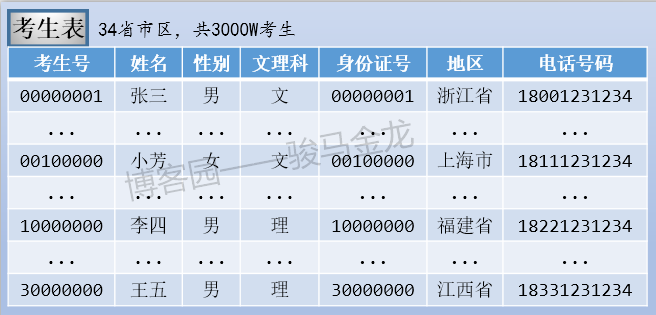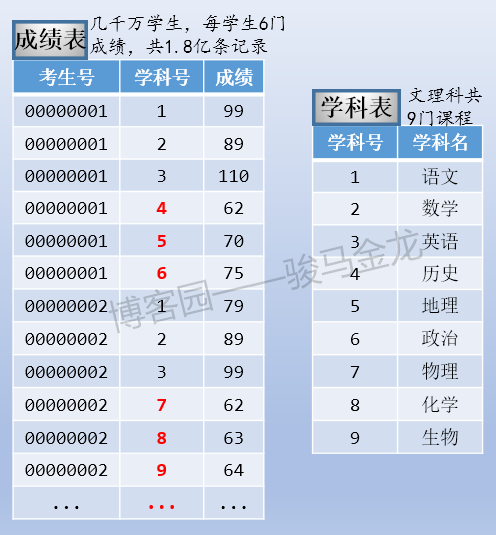# 2.业务分库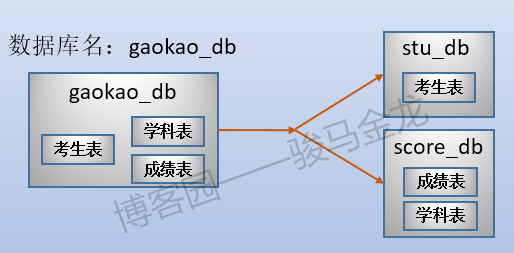stu_db和score_db可以放在同一数据库服务器上，也可以放在不同数据库服务器上，从而在整体上减轻系统的压力。但是，如果这两个库放在不同服务器上，因为跨数据库实例，将没法对stu_db和score_db中的表进行join操作。

# 3.垂直切分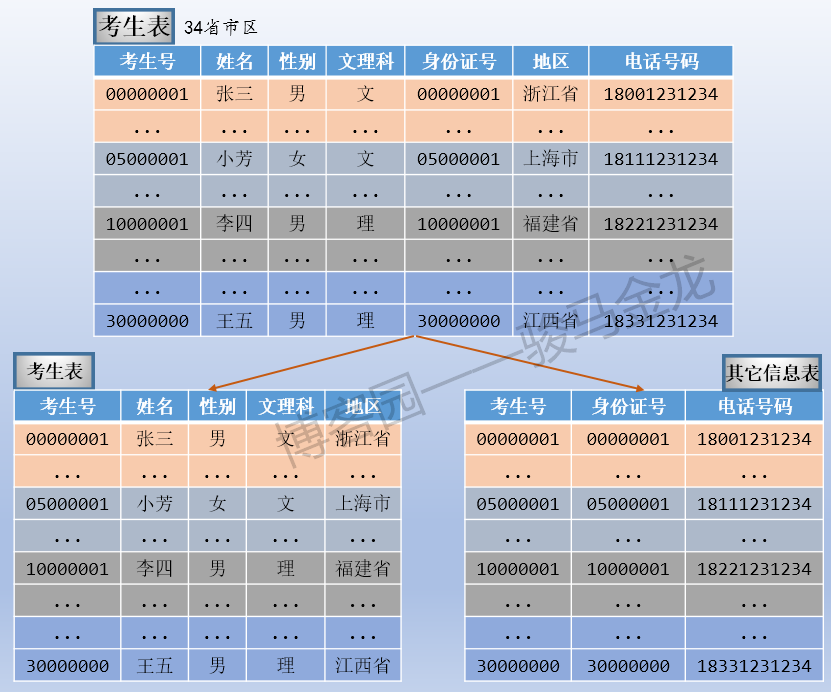# 4.水平切分

1. 直接按字段条件切分
2. 取模后切分
3. 按月份、季度、年份切分，或者称之为按范围切分

## 4.1 按字段条件进行切分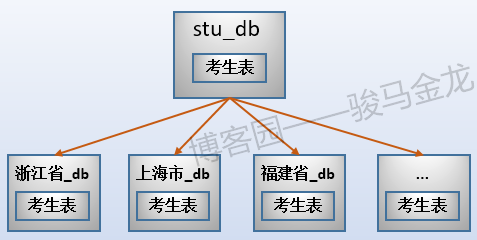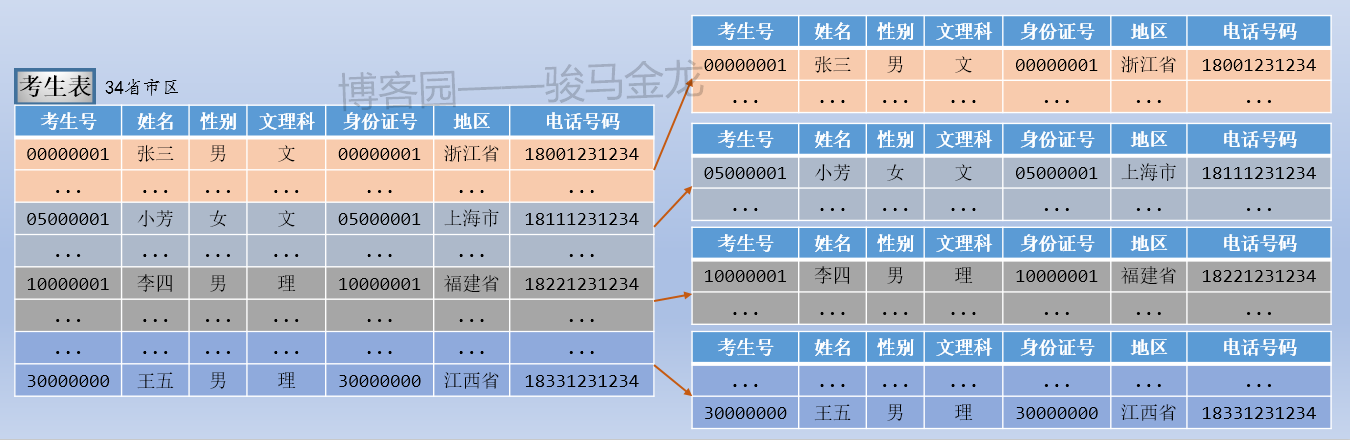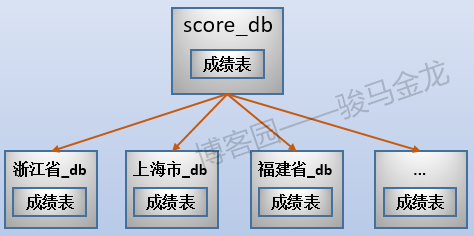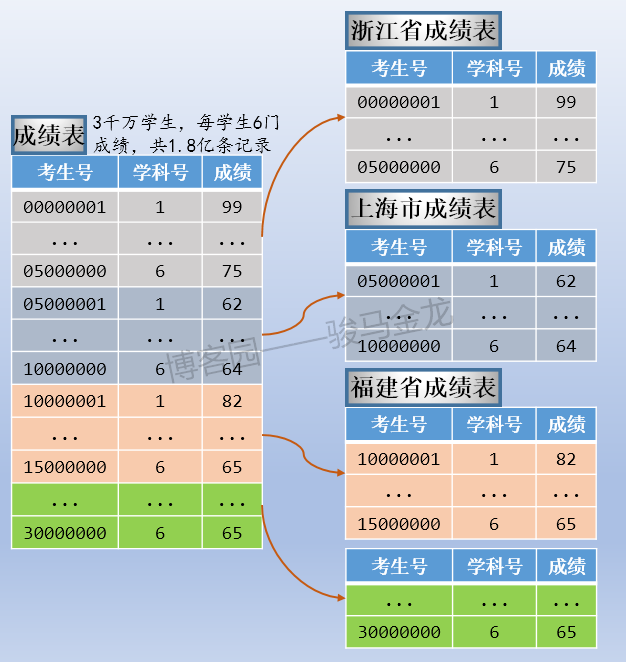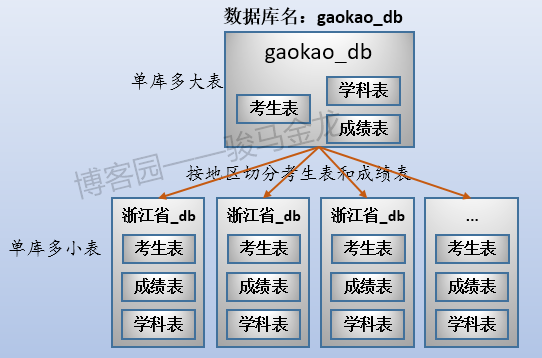## 4.2 按范围进行切分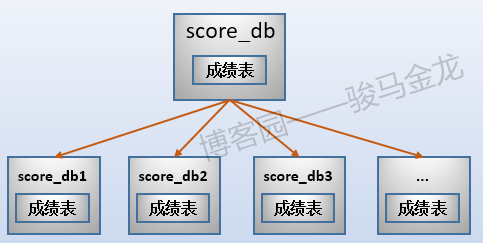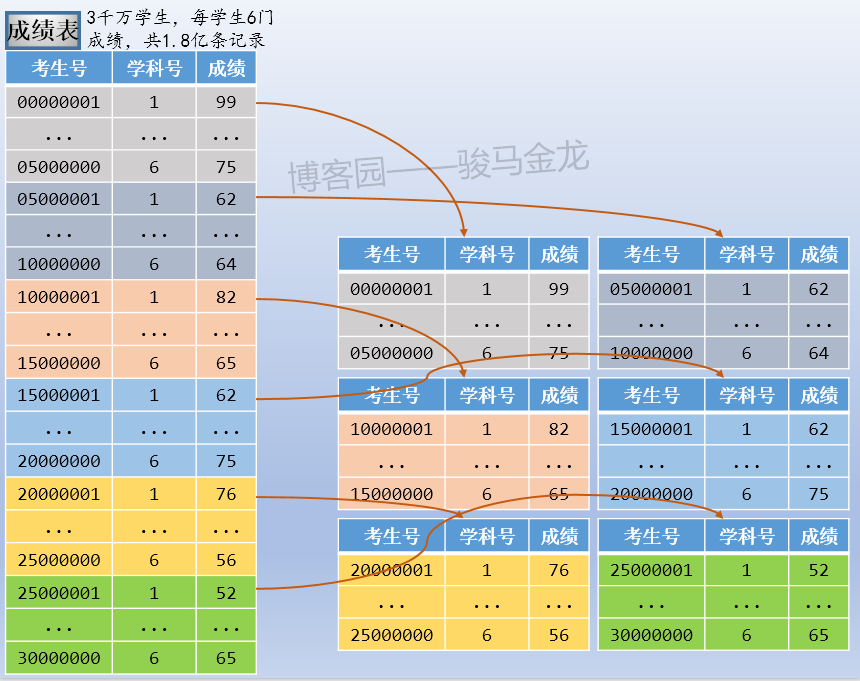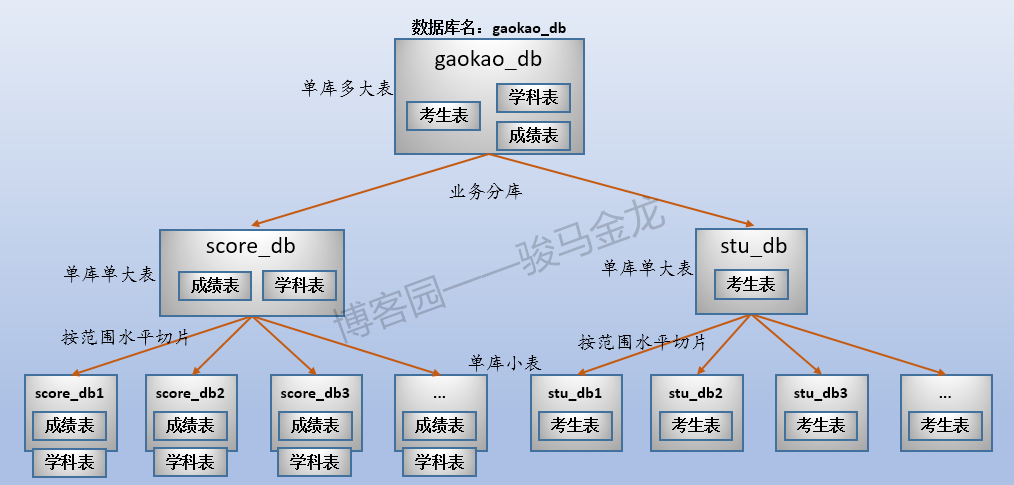## 4.3 取模切分

00000001 % 6 = 1   --> 放进stu_1库
00000002 % 6 = 2   --> 放进stu_2库
00000003 % 6 = 3   --> 放进stu_3库
00000004 % 6 = 4   --> 放进stu_4库
00000005 % 6 = 5   --> 放进stu_5库
00000006 % 6 = 0   --> 放进stu_0库
...
00000101 % 6 = 5   --> 放进stu_5库
00000102 % 6 = 0   --> 放进stu_0库
00000103 % 6 = 1   --> 放进stu_1库
00000104 % 6 = 2   --> 放进stu_2库
00000105 % 6 = 3   --> 放进stu_3库
00000106 % 6 = 4   --> 放进stu_4库
...


# 5.数据库分区

1. 分区方式和水平切片是类似的，分区方式也和水平切片方式类似，如范围切片，取模切片等
2. 数据库分区是数据库自身的特性，切片则是外部强制手段控制完成的
3. 数据库分区无法将分区跨库，更不能跨数据库服务器，但能保存在不同数据文件从而放置在不同存储设备上
4. 数据库分区是数据库的特性，数据完整性、一致性等实现起来很方便，这一切都是数据库自身保证的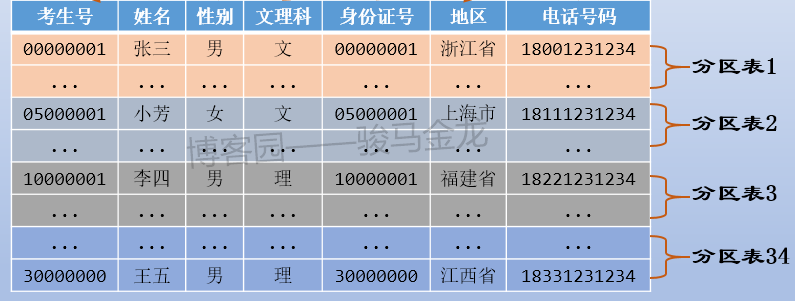# 6.分库、分表带来的问题

1.分库分表本身的复杂性

2.分库分表让数据库系统架构变得复杂

3.跨节点join问题

4.扩容和数据迁移艰难

• 扩容到多少节点比较满足自己的期望。
• 扩容时，哪些数据需要从旧节点清洗掉，哪些数据需要从旧节点迁移到新节点。
• 如何实现在线迁移。

  扩容前         扩容后
0 % 4 = 0     0 % 6 = 0
1 % 4 = 1     1 % 6 = 1
2 % 4 = 2     2 % 6 = 2
3 % 4 = 3     3 % 6 = 3
4 % 4 = 0     4 % 6 = 4   -> 从0节点迁移到4节点
5 % 4 = 1     5 % 6 = 5   -> 从1节点迁移到5节点
6 % 4 = 2     6 % 6 = 0   -> 从2节点迁移到0节点
7 % 4 = 3     7 % 6 = 1   -> 从3节点迁移到1节点
8 % 4 = 0     8 % 6 = 2   -> 从0节点迁移到2节点
9 % 4 = 1     9 % 6 = 3   -> 从1节点迁移到3节点
10 % 4 = 2    10 % 6 = 4  -> 从2节点迁移到4节点
11 % 4 = 3    11 % 6 = 5  -> 从3节点迁移到5节点
12 % 4 = 0    12 % 6 = 0
13 % 4 = 1    13 % 6 = 1
14 % 4 = 2    14 % 6 = 2
15 % 4 = 3    15 % 6 = 3
16 % 4 = 0    16 % 6 = 4  -> 从0节点迁移到4节点


  扩容前         扩容后
0 % 4 = 0     0 % 8 = 0
1 % 4 = 1     1 % 8 = 1
2 % 4 = 2     2 % 8 = 2
3 % 4 = 3     3 % 8 = 3
4 % 4 = 0     4 % 8 = 4  -> 从0节点迁移到4节点
5 % 4 = 1     5 % 8 = 5  -> 从1节点迁移到5节点
6 % 4 = 2     6 % 8 = 6  -> 从2节点迁移到6节点
7 % 4 = 3     7 % 8 = 7  -> 从3节点迁移到7节点
8 % 4 = 0     8 % 8 = 0
9 % 4 = 1     9 % 8 = 1
10 % 4 = 2    10 % 8 = 2
11 % 4 = 3    11 % 8 = 3
12 % 4 = 0    12 % 8 = 4  -> 从0节点迁移到4节点
13 % 4 = 1    13 % 8 = 5  -> 从1节点迁移到5节点
14 % 4 = 2    14 % 8 = 6  -> 从2节点迁移到6节点
15 % 4 = 3    15 % 8 = 7  -> 从3节点迁移到7节点
16 % 4 = 0    16 % 8 = 0


1. 加入新节点。
2. 将业务写入过程按照旧规则和新规则同时写到新旧节点(业务双写)。例如4节点扩容到6节点时，id=2000的数据(假设之前没有该数据)将同时写入到0节点和2节点，id=2003将同时写入3节点和5节点。
3. 迁移旧数据。
4. 应用新规则，将新节点向外提供服务。
5. 清洗旧数据。

posted @ 2018-07-30 00:38  骏马金龙  阅读(13261)  评论(3编辑  收藏